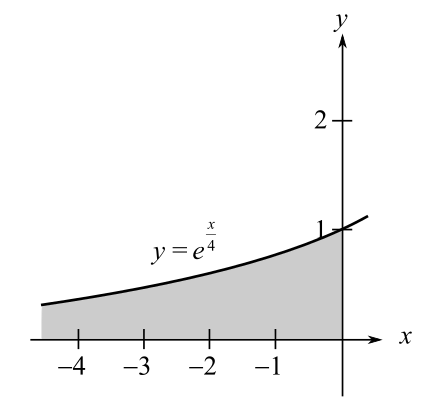Chapter 6.4, Problem 22E### Calculus: An Applied Approach (Min...

10th Edition
Ron Larson
ISBN: 9781305860919

#### Solutions

Chapter
Section### Calculus: An Applied Approach (Min...

10th Edition
Ron Larson
ISBN: 9781305860919
Textbook Problem
1 views

# Area of a Region In Exercises 21-26, find the area of the unbounded shaded region. y = e x / 4To determine

To calculate: The area of the unbounded shaded region for the function y=ex4:Explanation

Given Information:

The provided function is, y=ex4 and the graph is:

Formula used:

The area between two graphs:

If f(x)g(x) for all x in [a,b] (so that the graph of f doesn’t move below that of g), then the area of the region between the graphs of f and g and between x=a and x=b is given by:

A=ab[f(x)g(x)dx]

From definition of improper integral.

af(x)dx=limbabf(x)dx

Also, the expression for the integration of an exponential is as follows:

eaxdx=eaxa+C; where a0.

Calculation:

Here, the two functions are f(x)=ex4 and g(x)=0 and the graph is going from  to 0

### Still sussing out bartleby?

Check out a sample textbook solution.

See a sample solution

#### The Solution to Your Study Problems

Bartleby provides explanations to thousands of textbook problems written by our experts, many with advanced degrees!

Get Started

#### Find the mean for the following set of scores: 2, 7, 9, 4, 5, 3, 0, 6

Essentials of Statistics for The Behavioral Sciences (MindTap Course List)

#### For y = (x3 + 1)2, y = _____. a) 2x(x3 + 1) b) 6x(x3 + 1) c) 6x2(x3 + 1) d) 3x2(x3 + 1)

Study Guide for Stewart's Single Variable Calculus: Early Transcendentals, 8th

#### What is the goal of a single-case experimental research design?

Research Methods for the Behavioral Sciences (MindTap Course List)